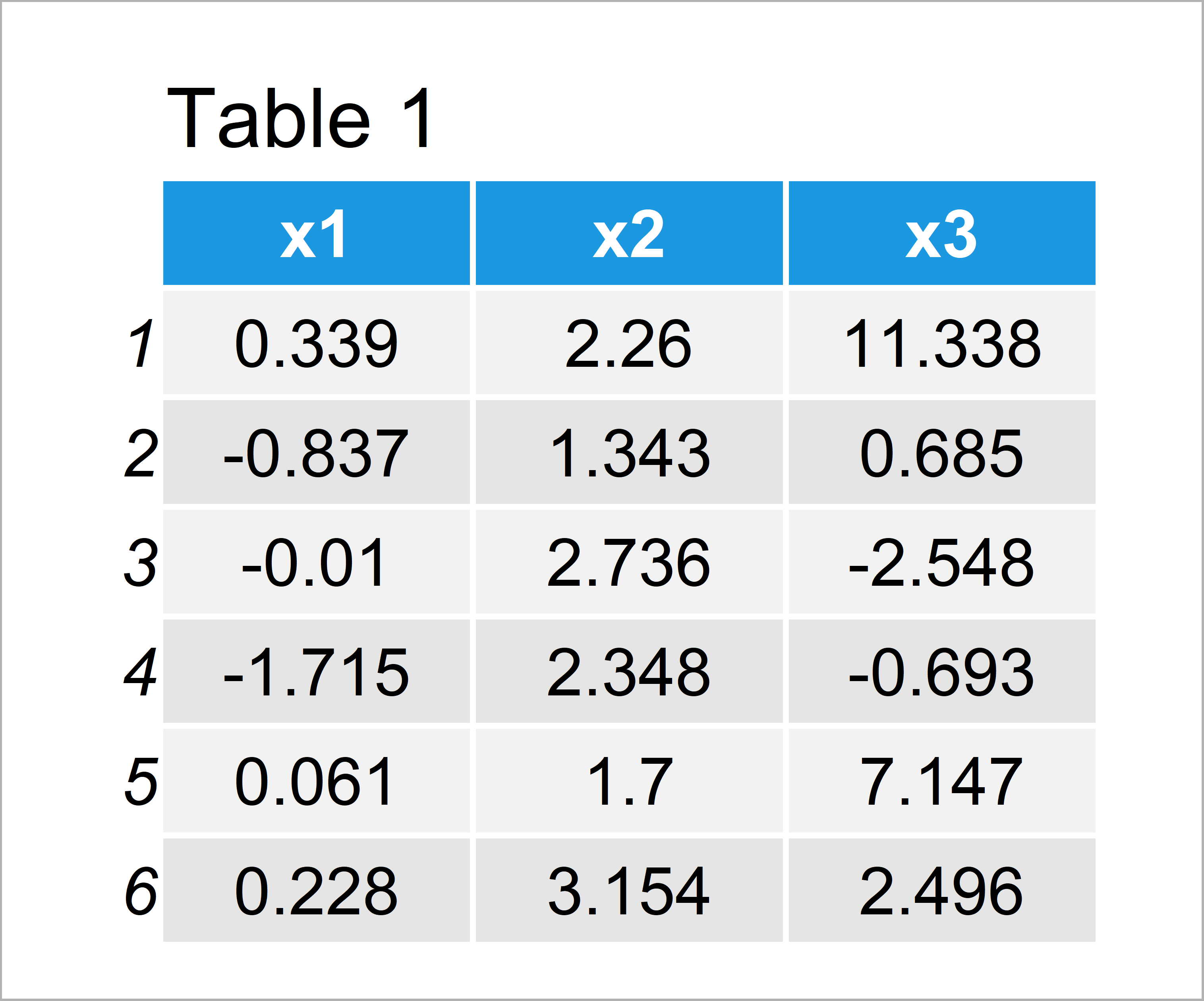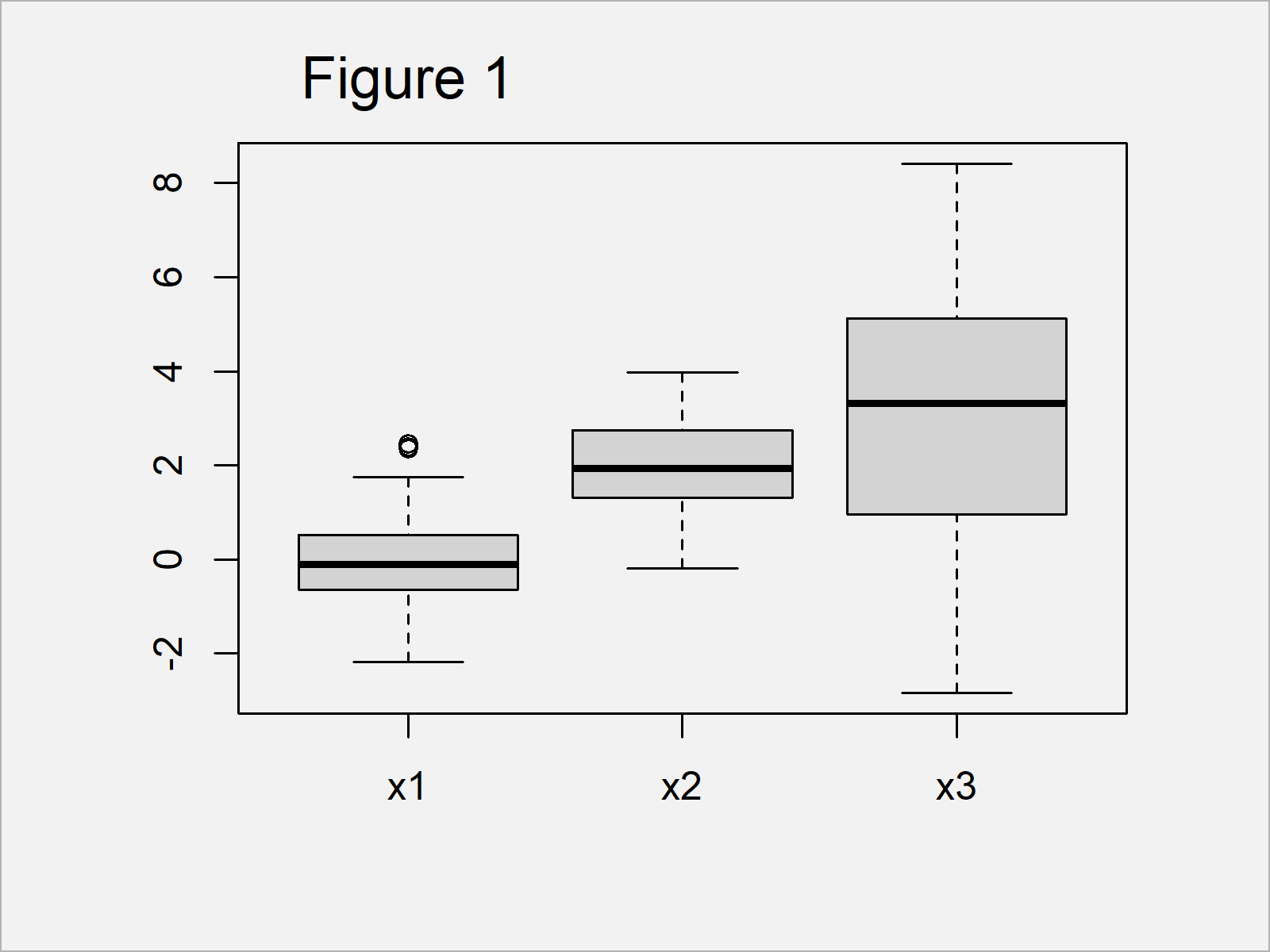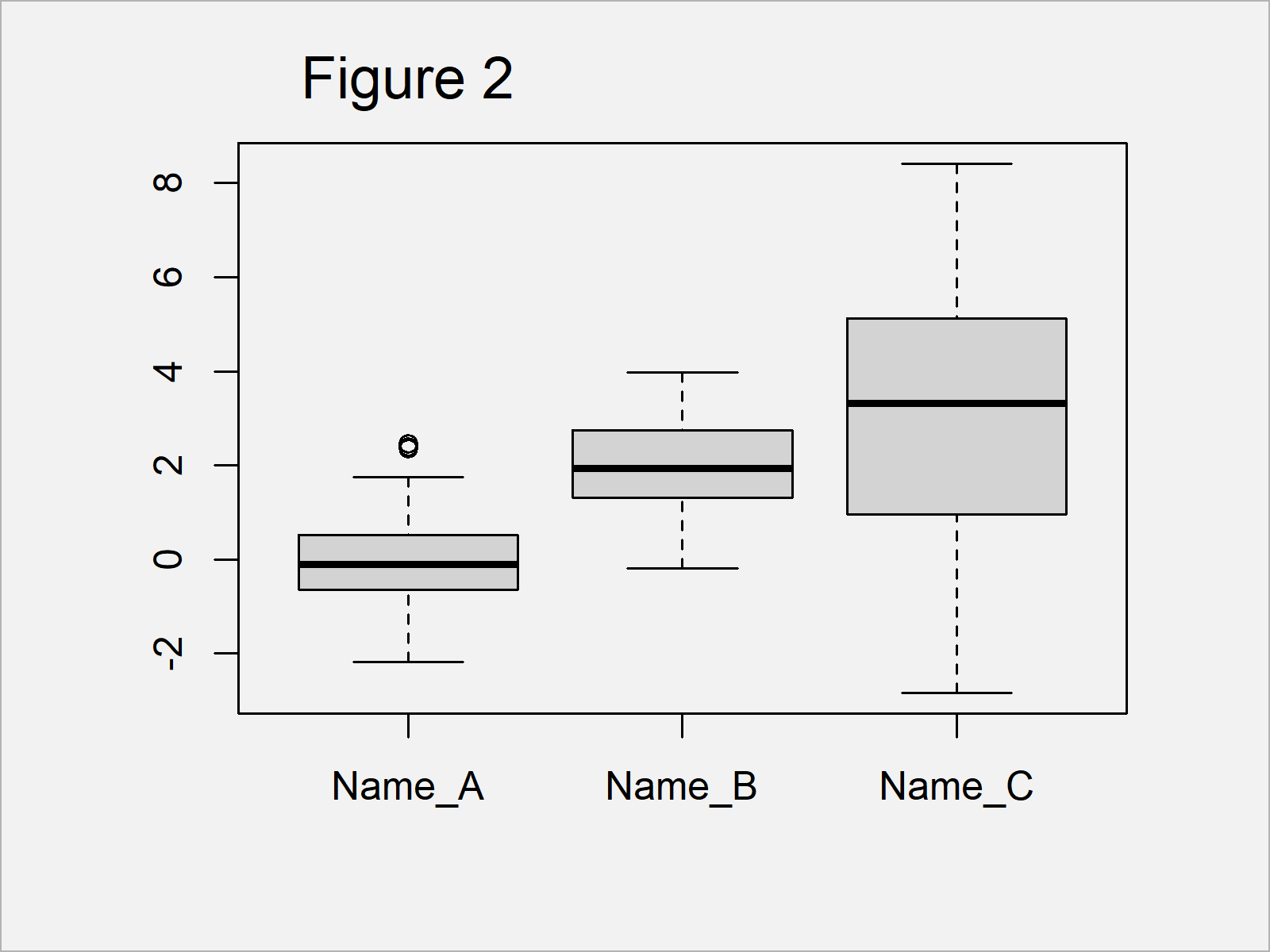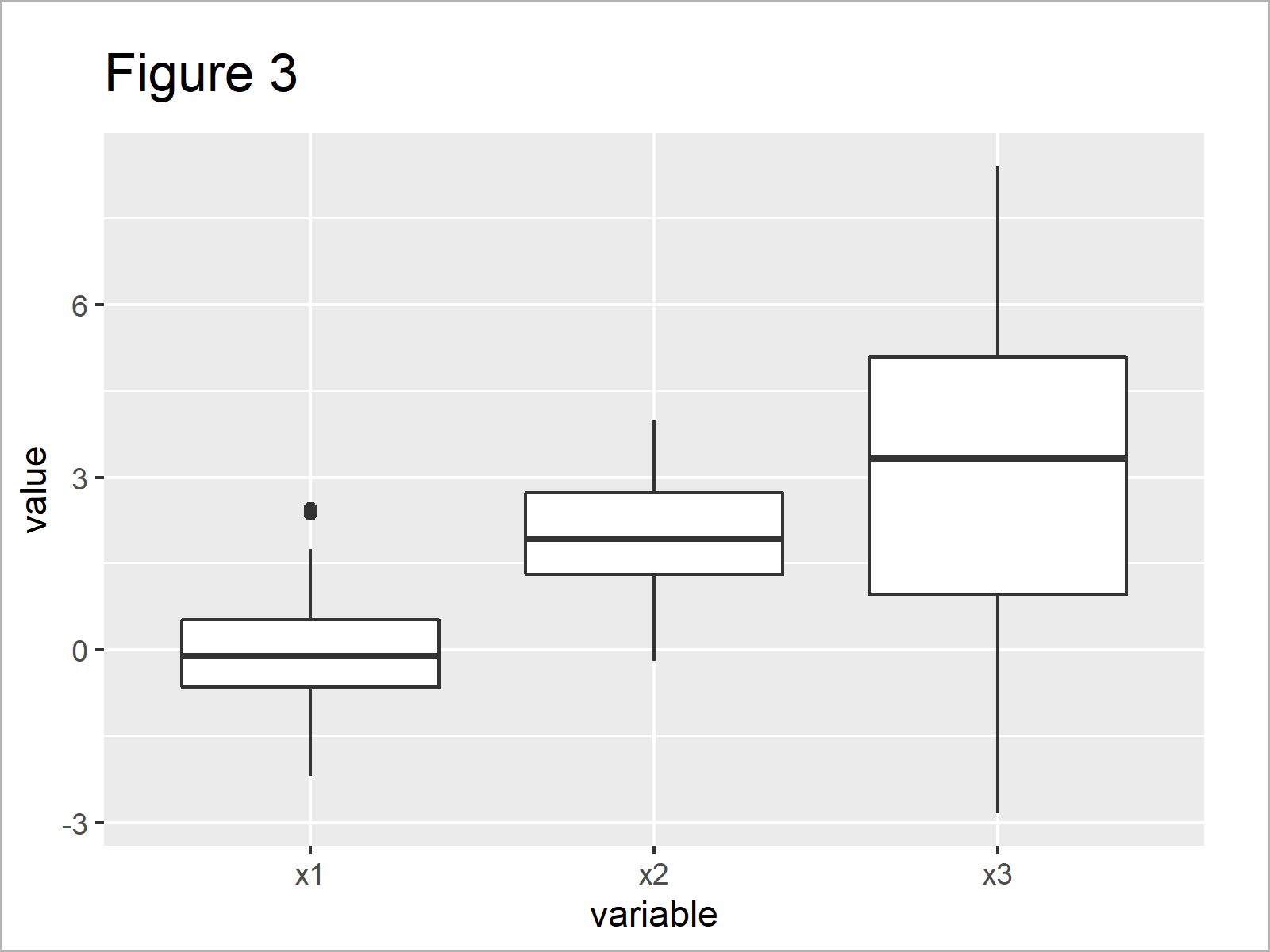# Change Axis Labels of Boxplot in R (2 Examples)

In this article, I’ll illustrate how to rename the x-axis labels of a boxplot in the R programming language.

The article will consist of these contents:

So let’s start right away…

## Example Data & Default Plot

The following data will be used as basement for this R programming tutorial:

```set.seed(9736425)                                 # Create example data
data <- data.frame(x1 = rnorm(100),
x2 = rnorm(100, 2),
x3 = rnorm(100, 3, 3))As you can see based on Table 1, our example data is a data frame consisting of 100 rows and three columns.

## Example 1: Change Axis Labels of Boxplot Using Base R

In this section, I’ll explain how to adjust the x-axis tick labels in a Base R boxplot.

Let’s first create a boxplot with default x-axis labels:

`boxplot(data)                                     # Boxplot in Base R`The output of the previous syntax is shown in Figure 1 – A boxplot with the x-axis label names x1, x2, and x3.

We can rename these axis labels using the names argument within the boxplot function:

```boxplot(data,                                     # Change labels of boxplot
names = c("Name_A",
"Name_B",
"Name_C"))```In Figure 2 you can see that we have plotted a Base R box-and-whisker graph with the axis names Name_A, Name_B, and Name_C.

## Example 2: Change Axis Labels of Boxplot Using ggplot2 Package

It is also possible to modify the axis labels of a ggplot2 boxplot.

As a first step, we have to reshape our input data frame from wide to long format using the reshape2 package.

First, we need to install and load the reshape2 package:

```install.packages("reshape2")                      # Install reshape2 package

Next, we can apply the melt function to transform our data frame:

```data_long <- melt(data)                           # Reshape dataIn Table 2 you can see that we have created a data frame in long format.

As next step, we need to install and load the ggplot2 package:

```install.packages("ggplot2")                       # Install ggplot2 package

Let’s draw a ggplot2 boxplot with default axis specifications:

```ggplot(data_long, aes(variable, value)) +         # Boxplot in ggplot2
geom_boxplot()```As shown in Figure 3, we have managed to create a ggplot2 boxplot using the previously shown R code.

The simplest solution for changing the x-axis labels is that we change the label names in our long data frame. Have a look at the R code below:

```data_long_labels <- data_long                     # Duplicate data
levels(data_long_labels\$variable) <- c("Name_A",  # Relevel factor labels
"Name_B",
"Name_C")```

After executing the previous R code, our variable names were changed to Name_A, Name_B, and Name_C.

Next, we can use this updated data frame to create another boxplot with the ggplot2 package:

```ggplot(data_long_labels, aes(variable, value)) +  # Boxplot with updated labels
geom_boxplot()```Figure 4 illustrates the output of the previous R programming syntax: A ggplot2 boxplot with renamed axis tick labels.

## Video & Further Resources

I have recently published a video on my YouTube channel, which illustrates the R codes of this article. You can find the video below.

Please accept YouTube cookies to play this video. By accepting you will be accessing content from YouTube, a service provided by an external third party.If you accept this notice, your choice will be saved and the page will refresh.

Furthermore, you might want to read the other articles of my website. You can find a selection of related tutorials below.

In this R tutorial you have learned how to modify boxplot axis labels. If you have further comments and/or questions, don’t hesitate to let me know in the comments section below.

Subscribe to the Statistics Globe Newsletter